Study S4 Mathematics Sets: Venn Diagrams, Intersections & Union - Geniebook# Sets: Venn Diagrams, Intersections & Union

In this article, we will be learning about Set types and making Venn Diagrams as per the syllabus for Secondary 4 Mathematics class. We will study about

• Venn Diagrams.
• How to differentiate proper subsets from Subsets.
• The Union and Intersection of two Sets.

## Set Notations

 The statement $$p$$ is an element of set $$S$$ is represented by $$p \in S$$. The statement $$q$$ is not an element of set $$S$$ can be represented by $$q \notin S$$.## Equal Sets

If you have two sets, $$P$$ and $$Q$$, which are equal, they would have to contain the same elements

Equal sets can be represented by $$P=Q$$.

However, the order of elements can be different.

Example:

Given $$P$$ = {1, 2, 3, 4} and $$Q$$ = {2, 4, 1, 3}.

Since they have the same elements and the number of elements is also the same, hence $$P=Q$$.

## Empty Set

A set $$P$$ is empty if it does not contain any elements at all.

Example:

$$P$$ = { } or Ø

$$P$$ is called an empty set or a null set.

## Universal Set And Complement Of A Set

 A universal set can be expressed by . The set of elements in but not in $$A$$ is called the Complement of a Set, denoted by $$A'$$. For example, in the given image, the elements 2, 4, 6, 8, 10 represent the complement of Set, $$A'$$.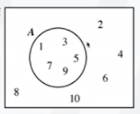## Proper Subset

Question 1:

Consider set $$P$$ = {2, 4, 6, 8, 10, 12} and $$Q$$ = {4, 6, 8}. How can we draw a Venn diagram to represent the sets?

Solution:

To draw the Venn diagram, we need to draw the Universal Set first. There are 2 sets, so we will have to draw 2 large circles.

 Notice that the elements {4, 6, 8} are common in $$Q$$ as well as $$P$$.  It means that we would have to draw a larger circle to represent $$P$$ and a smaller circle to represent $$Q$$.  Inside of $$Q$$, we have {4, 6, 8} because these elements are common. They are in $$Q$$ and also in $$P$$.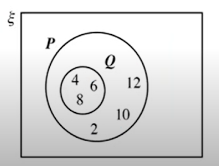There are 3 elements {2,10,12}, which are in $$P$$ but not in $$Q$$.

So, we will write them outside of $$Q$$ but still within $$P$$.

Consider a scenario where every element of $$Q$$ is also an element of $$P$$, but $$Q \neq P$$.

Therefore, we write that $$Q$$ is a proper subset of $$P$$

That means $$Q$$ is a smaller set as compared to $$P$$, and they share common elements. They are not the same, as there are 3 elements {2, 10, 12}, which are in $$P$$ but not in $$Q$$. The symbol to represent this statement is $$Q\subset P$$.

## Subset

What is the difference between a proper subset and a subset?

Let’s say we have a set $$X = {1, 3, 5}$$ .

If $$Y$$ is a proper subset of $$X$$, what are the possible elements that are in $$Y$$?

$$Y$$ could have elements {1}, {3} or {5}.

Instead of having just one common element, it could also have 2 common elements. It could be {1, 3}, {1, 5}, or {3, 5}.

It could also have no common elements - a null set Ø.

These are the possible elements of $$Y$$ if $$Y \subset X$$

If we are to call $$Z$$ a regular subset of X, what are the possible elements in $$Z$$?

It would be the same. We could have {1}, {3}, {5}, {1, 3}, {1, 5}, {3, 5}, or { }.

There is one other possibility that could apply to subsets but not to proper subsets.

The last possibility is that it shares all the exact elements as $$X$$. So, if $$Z\subseteq X$$, it means it could have the same elements as $$Z$$, meaning that $$Z=X$$

But for proper subsets, the 2 sets strictly cannot be equal.

So, for a proper subset, we will use symbol, and for a subset, we will use $$\subseteq$$.

## Proper Subset vs. Subset

Let's talk about the differences between proper subset and subset.

All the elements in the subset and proper subset are also elements of the bigger set.

If $$Z$$ is a subset, $$Z$$ and $$X$$ may be equal. For a proper subset, $$Y$$ is strictly not equal to $$X$$. That means they must not have the same elements.

The symbol used to represent a proper subset is $$Y\subset X$$ and $$Z\subseteq X$$ for a subset. The line below the subset symbol means that $$Z$$ and $$X$$ can be equal.

 So, a proper subset in a Venn diagram would look like this, where $$X$$ is the bigger set and $$Y$$ is the smaller set. There are some common elements inside and some elements outside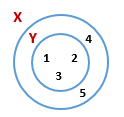For a regular subset, you have 2 sets with some common elements inside and uncommon elements outside.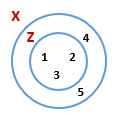Or you could have 2 sets, $$Z$$ and $$X$$, with all the common elements inside and no uncommon elements. In the second case, $$Z=X$$. Both cases are possible.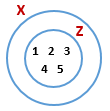### Example 3:

It is given that

$$A$$ = {$$x$$ : $$x$$ is a multiple of 3 such that 10 ≤ $$x$$ ≤ 25} and

$$B$$ = {$$x$$ : $$x$$ is a multiple of 12 such that 10 ≤ $$x$$ ≤ 30}.

1. List all the elements in $$A$$ and in $$B$$ in set notation.
2. Draw a Venn diagram to represent the sets of $$A$$ and $$B$$.
3. Is $$B$$ a proper subset of $$A$$? Explain your answer.

Solution:

 1 $$A =$$ {12, 15, 18, 21, 24} $$B =$$ {12, 24}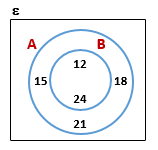2 To draw a Venn diagram, first, draw the universal set with a bigger circle to represent Set $$A$$ and a smaller circle for Set $$B$$. The common elements are 12, 24. Every other element of Set $$A$$ should be out of Set $$B$$ but within Set $$A$$. 3 Yes, $$B\subset A$$. All elements in Set $$B$$ are also in Set $$A$$ and $$B\neq A$$, fulfilling the criteria for a proper subset.

### Intersection Of Two Sets

Let’s understand this concept through an example.

### Question 2:

Consider the sets $$M= \{3, 6, 9, 12, 15\}$$ and $$N= \{6, 12, 18, 24, 36\}$$

How can we draw a Venn diagram to represent the sets?

Solution:

 The common elements between the sets are {6, 12}. The Venn diagram for the sets would be as shown. The common area consisting of common elements between the sets is known as the Intersection. The uncommon elements remaining in the sets will be placed outside the intersection. The elements common to both sets $$M$$ and $$N$$ lie in the intersection of $$M$$ and $$N$$. This can be represented by $$M\cap N = \{6, 12\}$$.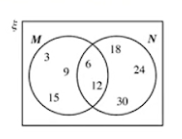### Question 3:

Consider Set $$C$$ = {1, 3, 5, 7, 9} and $$D$$ = {0, 2, 4, 6, 8}

How can we draw a Venn diagram to represent the sets?

Solution:

 Do $$C$$ and $$D$$ have any common elements? No. This is what is called Disjoint sets, which do not share any common elements or intersections. This can be represented as $$C\cap D$$ = { } or Ø. You can also represent disjoint sets in a Venn diagram with an empty intersection.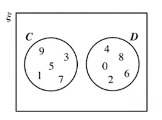### Example 4:

It is given that

$$G$$ = {$$x$$ : $$x$$ is a positive integer and a factor of 20} and

$$H$$ = {$$x$$ : $$x$$ is a single digit prime number}.

A. List all the elements in $$G$$ and in $$H$$ in set notation.

B. Find $$G\cap H$$.

C. Draw a Venn diagram to represent the sets $$G$$ and $$H$$.

Solution:

 A. $$G = \{1, 2, 4, 5, 10, 20\}$$ $$H= \{2, 3, 5, 7\}$$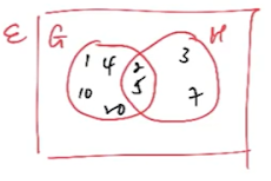B. The common elements are $$G\cap H = \{2, 5\}$$ C. Using elements of $$G$$, $$H$$, and $$G\cap H$$

### Question 4:

Consider the sets $$R = \{1, 4, 9, 16, 25\}$$ and $$S = \{4, 8, 12, 16\}.$$

How can we draw a Venn diagram to represent the sets?

Solution:

 The common elements between $$R$$ and $$S$$ are $$\{4, 16\}$$. In intersections, we are interested in finding the common part. But in Union, we're interested in everything. Everything inside the 2 circles refers to the Union.  The intersection is like an overlap, but the union is like a marriage. If we list all the elements which are in $$R$$ or in $$S$$ in ascending order, we will get $$\{1, 4, 8, 9, 12, 16, 25\}.$$ The common elements don't need to be written twice. This is called the Union of $$R$$ and $$S$$.  To express using symbols, we use  $$R\cup S$$, which is a set of its own.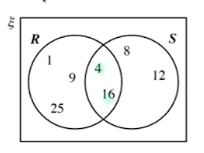Example 5:

It is given that

$$​ A = \{x : x \text{ is a positive integer less than 30 and is a multiple of 6} \} ​$$ and

$$​ B = \{x :x \text { is a positive integer and is a factor of 12} \}. ​$$

1. List all the elements in $$A$$ and in $$B$$ in set notation.

2. Draw a Venn diagram to represent the sets  $$A$$ and $$B$$.

3. From the Venn diagram, find $$A\cup B$$.

Solution:

 1 $$A= \{6, 12, 18, 24\}$$  $$B = \{1, 2, 3, 4, 6, 12\}$$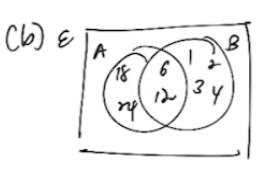2 Take note of the common elements. $$A\cap B = {6, 12}$$ 3 Use the Venn diagram to find the union. $$A\cup B = \{1, 2, 3, 4, 6, 12, 18, 24\}.$$ It is just all the elements together.

## Conclusion

In this article, we learned the concepts of subsets and proper subsets, as well as how to differentiate between them using Venn diagrams. We talked about set notations and the concepts of union and intersection of sets. The details covered are as per the Secondary 4 Mathematics grade.

Continue Learning
Sets: Venn Diagrams, Intersections & Union Probability of Combined Events
Statistical Data Analysis Matrices
VectorsPrimaryPrimary 1Primary 2Primary 3Primary 4Primary 5Primary 6SecondarySecondary 1Secondary 2Secondary 3Secondary 4PhysicsChemistryMathsE-Maths
Sets: Venn Diagrams, Intersections & Union
Probability of Combined Events
Statistical Data Analysis
Matrices
VectorsA-Maths